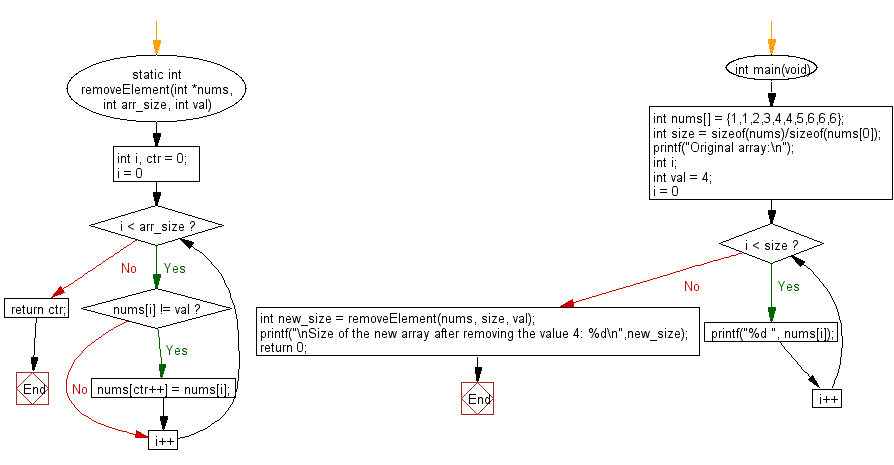﻿ C Program: Array length, remove instances of a value# C Exercises: Remove all instances of a given value in a given array of integers and return the length of the new array

## C Programming Practice: Exercise-13 with Solution

Write a C programming to remove all instances of a given value in a given array of integers and return the length of the new array.

C Code:

``````#include <stdio.h>
#include <stdlib.h>

static int removeElement(int *nums, int arr_size, int val)
{
int i, ctr = 0;
for (i = 0; i < arr_size; i++) {
if (nums[i] != val) {
nums[ctr++] = nums[i];
}
}
return ctr;
}

int main(void)
{
int nums[] = {1,1,2,3,4,4,5,6,6,6};
int size = sizeof(nums)/sizeof(nums);
printf("Original array:\n");
int i;
int val = 4;
for (i = 0; i < size; i++) {
printf("%d ", nums[i]);
}
int new_size = removeElement(nums, size, val);
printf("\nSize of the new array after removing the value 4: %d\n",new_size);
return 0;
}
``````

Sample Output:

```Original array:
1 1 2 3 4 4 5 6 6 6
Size of the new array after removing the value 4: 8
```

Pictorial Presentation:Flowchart:## C Programming Code Editor:

What is the difficulty level of this exercise?

Test your Programming skills with w3resource's quiz.

﻿

## C Programming: Tips of the Day

Maximum value of int:

In C:

```#include <limits.h>
then use
int imin = INT_MIN; // minimum value
int imax = INT_MAX;```

or

```#include <float.h>

float fmin = FLT_MIN;  // minimum positive value
double dmin = DBL_MIN; // minimum positive value

float fmax = FLT_MAX;
double dmax = DBL_MAX;```

Ref : https://bit.ly/3fi8yk9